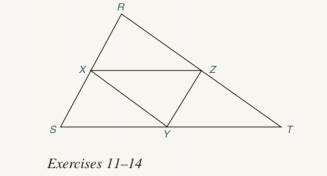Chapter 4.2, Problem 14E### Elementary Geometry for College St...

6th Edition
Daniel C. Alexander + 1 other
ISBN: 9781285195698

#### Solutions

Chapter
Section### Elementary Geometry for College St...

6th Edition
Daniel C. Alexander + 1 other
ISBN: 9781285195698
Textbook Problem
1 views

# In Exercises 11 to 14 , assume that X , Y , and Z are midpoints of the sides of ∆ R S T .For Exercise 12 to 14, see the figure for Exercise 11.If the perimeter (sum of the lengths of all three sides) of ∆ X Y Z is 12.7, what is the perimeter of ∆ R S T ?To determine

To find:

The perimeter of the RST.

Explanation

Approach:

The line segment that joins the midpoints of two sides of a triangle is parallel to the third side and has a length equal to one half the length of the third side.

Calculation:

Given,

The perimeter of XYZ=12.7 units.

X, Y, Z are the midpoints of the sides of RST

By the application of the ‘theorem the line segment that joins the midpoints of two sides of a triangle is parallel to the third side and has a length equal to one half the length of the third side’, we have

XY=12RT

Multiply; by 2 on both sides,

2XY=2·12

### Still sussing out bartleby?

Check out a sample textbook solution.

See a sample solution

#### The Solution to Your Study Problems

Bartleby provides explanations to thousands of textbook problems written by our experts, many with advanced degrees!

Get Started

#### Solve the equations in Exercises 126. 1x211+1=0

Finite Mathematics and Applied Calculus (MindTap Course List)

#### The implied domain of is: (1, ∞) (−∞, 1) x ≠ 1 (−1, 1)

Study Guide for Stewart's Single Variable Calculus: Early Transcendentals, 8th

#### Given the Taylor Series , a Taylor series for ex/2 is:

Study Guide for Stewart's Multivariable Calculus, 8th